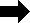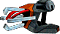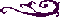PNTA FILE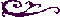3D Point Array - Level and Character File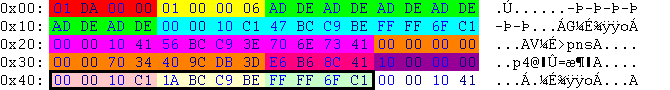HexTranslationMeaning
01 DA 00 0021800218-.PNTA
01 00 00 063level 3
00 00 10 C1-9.000000minimum z-coordinate of all packages below
47 BC C9 BE-0.394015minimum y-coordinate (height) of all packages below
FF FF 6F C1-14.999999minimum x-coordinate of all packages below
00 00 10 419.000000maximum z-coordinate of all packages below
56 BC C9 3E0.394015maximum y-coordinate (height) of all packages below
70 6E 73 4115.214462maximum x-coordinate of all packages below
00 00 00 000.000000z-coordinate of the center [ ((max. z-coord. - min. z-coord.) ÷ 2) + min z-coord. ]
00 00 70 340.000000...y-coordinate of the center [ ((max. y-coord. - min. y-coord.) ÷ 2) + min y-coord. ]
40 9C DB 3D0.107232x-coordinate of the center [ ((max. x-coord. - min. x-coord.) ÷ 2) + min x-coord. ]
E6 B6 8C 4117.589306distance from the center to each of the both points above
10 00 00 001616 packages follow (one package is edged in black)
Below follows the first package.
00 00 10 C1-9.000000z-coordinate of the first point
1A BC C9 BE-0.394013y-coordinate (height) of the first point
FF FF 6F C1-14.999999x-coordinate of the first point

The first image shows the light blue, lila and dark orange marked points of the table above. In my opinion these points are used to create the bounding box.

The second image gives you an overview of the packages. Every package contains the x- y- and z-coordinate of a point. In this example every point excists in duplicate, so that you can only see 8 points. These points are needed to built up the mesh (triangles) for a door.

Image number three is a fusion of the first and the second image.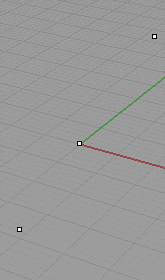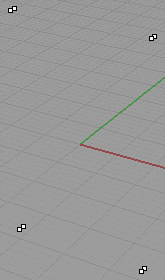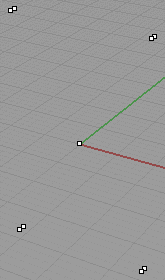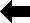Back to File Types